# NABARD Manager Quant Aptitude Mock Test 7

## 20 Questions MCQ Test NABARD Manager - Mock Tests & Previous Year Papers | NABARD Manager Quant Aptitude Mock Test 7

Description
Attempt NABARD Manager Quant Aptitude Mock Test 7 | 20 questions in 12 minutes | Mock test for Banking Exams preparation | Free important questions MCQ to study NABARD Manager - Mock Tests & Previous Year Papers for Banking Exams Exam | Download free PDF with solutions
QUESTION: 1

Solution:
QUESTION: 2

### The length of one diagonal of a rhombus is 80% of the other diagonal. The area of the rhombus is how many times the square of the length of the longer diagonal?

Solution: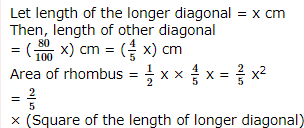QUESTION: 3

### If the selling price of 16 items is same as the cost price of 20 items, then there is a

Solution:

⇒  Let Selling price of 1 item = Rs.1.

⇒   Then, Selling price of 16 items = Rs.16

⇒  We are given  that cost price of 20 items = Rs.16.

⇒   C.P. of 1 item = 16/20 = Rs.0.8

⇒   Profit on every item = S.P.−C.P.= 1−0.8 = 0.2

⇒   Gain percentage =
QUESTION: 4
A sample of 50 litres of glycerine is found to be adulterated to the extent of 20%. How much pure glycerine should be added to it so as to bring down the percentage of impurity to 5%?
Solution:
QUESTION: 5

If 40% of a number is added to an other number then it becomes 125% of itself.What will be the ratio of first and second number?

Solution:

Let first number is x & second no. is y
QUESTION: 6
Choose the correct alternative that will continue the same pattern and fill in the blank.
(1/9), (1/3), 1, ____ , 9
Solution: This is a multiplication series; each number is 3 times the previous number.
QUESTION: 7

The average age of a husband and his wife was 23 years at the time of their marriage. After five years they have a one-year old child. The average age of the family now is :

Solution:

Sum of the present ages of husband, wife and child = ((23x2) + (5 x 2)) + 1 = 57 years
Required average age of the family now = 57 3 = 19 years.

QUESTION: 8
If three squares are chosen at random from the squares 8*8 chessboard, then find the probability that exactly two of them are of the same colour ?
Solution:
QUESTION: 9
If A:B=7:9 and B:C=3:5,then A:B:C is
Solution:
QUESTION: 10
What will be the total simple interest on Rs 80,000 at the rate of 8% per annum at the end of 6 yrs?
Solution:
QUESTION: 11

A contractor undertook to build a road in 100 days. He employed 110 men. After 45 days, he found that only 1/4 could be built. In order to complete the work in time, how many more men should be employed?

Solution: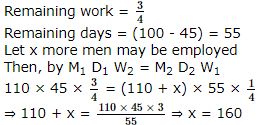QUESTION: 12

The cost of painting the whole surface area of a cube at the rate of 13 paise per sq. cm is Rs. 343.98. Then the volume of the cube is :

Solution:
QUESTION: 13

In the following questions two equations numbered I and II are given. You have to solve both the equations and give answer.

I. x2 + 28 = 11 x
II. 2y2 + 18 = 12y

Solution:

I. x2 - 11x + 28 = 0
⇒ x2 - 7x - 4x + 28 = 0
⇒ x(x - 7) - 4(x - 7) = 0
⇒ x = 7, 4
Also,
II. 2y2 + 12y + 18 = 0
⇒ 2y2 + 6y + 6y + 18 = 0
⇒ 2y(y + 3) + 6(y + 3) = 0
⇒ y = -3, -3
Hence, x > y

QUESTION: 14

In the following questions two equations numbered I and II are given. You have to solve both the equations and give answer.

I. x2 + 5x = 7x + 8
II. y2 + 7y = 12y + 6

Solution:

I. x2 - 2x - 8 = 0
⇒ x2 - 4x + 2x - 8 = 0
⇒ x(x - 4) + 2(x - 4) = 0
⇒ x = 4, -2
Also,
II. y2 - 5y - 6 = 0
⇒ y2 - 6y + y - 6 = 0
⇒ y(y - 6) + 1(y - 6) = 0
⇒ x = 6, - 1
Hence, x < y

QUESTION: 15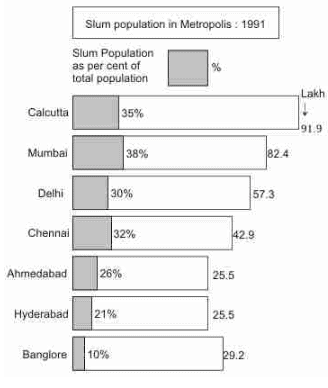The total slum population of Calcutta in 1991 was approximatey :

Solution:

Total slum population in Calcutta
= 91.9 � 35%
= 32 lakh (approximately)

QUESTION: 16The difference in the slum populations of Bangalore and Hyderabad was :

Solution:

Banglore = 2920000 � 10100 = 292000
Hyderabad = 2550000 � 21100 =535500
Difference = 535500 - 292000
= 243500 ≅ 2.43 lakh

QUESTION: 17

These questions are to be answered on the bases of the following pie chart which gives the marks scored by a student in an examination in five subjects- English,Hindi,Mathematics,Science and Social Science. Assuming that the total marks obtained for the examination are 540 answer these questions.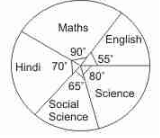The marks scored by the student in Hindi and Mathematics exceed the marks scored in English and Social Science by :

Solution:

% of marks obtained in Hindi and Maths = (90 + 70) = 160�
% of marks obtained in English and Social science = (65 + 55) = 120�
Difference = 160� - 120� = 40�
Now 360� = 540 marks
∴ 40� = 540/360� 40 = 60 marks

QUESTION: 18

These questions are to be answered on the bases of the following pie chart which gives the marks scored by a student in an examination in five subjects- English,Hindi,Mathematics,Science and Social Science. Assuming that the total marks obtained for the examination are 540 answer these questions.The subject in which the student scored 22.2% marks is :

Solution:

Hindi = 70/360� 100 = 19.44%
Science = 80/360 � 100 = 22.22%
Social Science = 65/360 � 100 = 18.05%
English = 55/360 � 100 = 15.28%

QUESTION: 19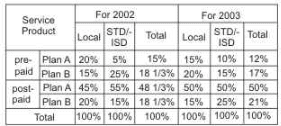Find the revenue from plan B for prepaid local services in year 2003 if the revenue for the plan A for the year 2002 was 600crores and there was a 100% increase in the overall revenue of the company from 2002 to 2003?

Solution:
QUESTION: 20Find the percentage by which the total revenue from postpaid STD/ISD services in 2003 is more as compared to that from postpaid local services in 2003.

Solution:Use Code STAYHOME200 and get INR 200 additional OFF Use Coupon Code